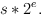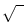Next: The data type real Up: Number Types and Linear Previous: Rational Numbers ( rational   Contents   Index

# The data type bigfloat ( bigfloat )

Definition

In general a bigfloat is given by two integers s and e where s is the significant and e is the exponent. The tuple (s, e) represents the real numberIn addition, there are the special bigfloat values NaN (not a number), pZero, nZero (= + 0, - 0), and pInf, nInf ( = +, -). These special values behave as defined by the IEEE floating point standard. In particular,,,+1 =,, ++ (-) = NaN and 0*= NaN.

Arithmetic on bigfloats uses two parameters: The precision prec of the result (in number of binary digits) and the rounding mode mode. Possible rounding modes are:

• TO_NEAREST: round to the closest representable value
• TO_ZERO: round towards zero
• TO_INF: round away from zero
• TO_P_INF: round towards +• TO_N_INF: round towards -• EXACT: compute exactly for +,-,* and round to nearest otherwise
Operations +, -, * work as follows. First, the exact result z is computed. If the rounding mode is EXACT then z is the result of the operation. Otherwise, let s be the significant of the result; s is rounded to prec binary places as dictated by mode. Operations / andwork accordingly except that EXACT is treated as TO_NEAREST.

The parameters prec and mode are either set directly for a single operation or else they are set globally for every operation to follow. The default values are 53 for prec and TO_NEAREST for mode.

#include < LEDA/numbers/bigfloat.h >

Creation

A bigfloat may be constructed from data types double, long, int and integer, without loss of accuracy. In addition, an instance of type bigfloat can be created as follows.

 bigfloat x(const integer& s, const integer& e) introduces a variable x of type bigfloat and initializes it to s*2e double x.to_double() returns the double value next to x (i.e. rounding mode is always TO_NEAREST). double x.to_double(bool& is_double) as above, but also returns in is_double whether the conversion was exact. double x.to_double(double& abs_err, rounding_modes m = TO_NEAREST) as above, but with more flexibility: The parameter m specifies the rounding mode. For the returned value d, we have | x - d| < = abserr. (abs_err is zero iff the conversion is exact and the returned value is finite.) double x.to_double(rounding_modes m) as above, but does not return an error bound. rational x.to_rational() converts x into a number of type rational. sz_t x.get_significant_length(void) returns the length of the significant of x. sz_t x.get_effective_significant_length(void) returns the length of the significant of x without trailing zeros. integer x.get_exponent(void) returns the exponent of x. integer x.get_significant(void) returns the significant of x. sz_t bigfloat::set_precision(sz_t p) sets the global arithmetic precision to p binary digits and returns the old value sz_t bigfloat::get_precision() returns the currently active global arithmetic precision sz_t bigfloat::set_output_precision(sz_t d) sets the precision of bigfloat output to d decimal digits and returns the old value sz_t bigfloat::set_input_precision(sz_t p) sets the precision of bigfloat input to p binary digits and returns the old value rounding_modes bigfloat::set_rounding_mode(rounding_modes m) sets the global rounding mode to m and returns the old rounding mode rounding_modes bigfloat::get_rounding_mode() returns the currently active global rounding mode output_modes bigfloat::set_output_mode(output_modes o_mode) sets the output mode to o_mode and returns the old output mode

A bigfloat x can be rounded by the call round(x,prec,mode,is_exact). The optional boolean variable is_exact is set to true if and only if the rounding operation did not change the value of x.

 integer to_integer(rounding_modes rmode = TO_NEAREST, bool& is_exact=bigfloat::dbool) returns the integer value next to x (in the given rounding mode) integer to_integer(const bigfloat& x, rounding_modes rmode, bool& is_exact) returns x.to_integer(...).

Operations

The arithmetical operators +, -, *, /, +=, -=, *=, /=, sqrt, the comparison operators <, < =, >, > = , =, ! = and the stream operators are available. Addition, subtraction, multiplication, division, square root and power are implemented by the functions add, sub, mul, div, sqrt and power respectively. For example, the call

 bool isNaN(const bigfloat& x) returns true if and only if x is in special state NaN bool isnInf(const bigfloat& x) returns true if and only if x is in special state nInf bool ispInf(const bigfloat& x) returns true if and only if x is in special state pInf bool isnZero(const bigfloat& x) returns true if and only if x is in special state nZero bool ispZero(const bigfloat& x) returns true if and only if x is in special state pZero bool isZero(const bigfloat& x) returns true if and only if ispZero(x) or isnZero(x) bool isInf(const bigfloat& x) returns true if and only if ispInf(x) or isnInf(x) bool isSpecial(const bigfloat& x) returns true if and only if x is in a special state int sign(const bigfloat& x) returns the sign of x. bigfloat abs(const bigfloat& x) returns the absolute value of x bigfloat ipow2(const integer& p) returns 2p integer ilog2(const bigfloat& x) returns the binary logarithm of abs(x), rounded up to the next integer. Precondition x ! = 0 integer ceil(const bigfloat& x) returns x, rounded up to the next integer integer floor(const bigfloat& x) returns x, rounded down to the next integer bigfloat sqrt_d(const bigfloat& x, sz_t p, int d) returns, with relative error < = 2-p but not necessarily exactly rounded to p binary digits string x.to_string(sz_t dec_prec=global_output_prec) returns the decimal representation of x, rounded to a decimal precision of dec_prec decimal places. bigfloat& x.from_string(string s, sz_t bin_prec=global_input_prec) returns an approximation of the decimal number given by the string s by a bigfloat that is accurate up to bin_prec binary digits ostream& ostream& os « const bigfloat& x writes x to output stream os istream& istream& is » bigfloat& x reads x from input stream is in decimal format